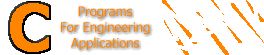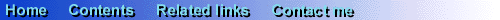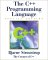The C++ Programming Language Special Edition

Plotting Polar Functions

Suppose you have a polar function in the form:

r = f ( ø )
where r is the length of line emerging from the origin to a point at an angle of ø.

and you need to plot it using a C program. All we need to do is to simply use the methods we used previously after converting polar coordinates into quadratic ones.

 Converting polar coordinates to quadratic coordinatesand vice versa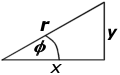The figure above shows the relationships between polar and quadratic coordinates. From this figure we can derive the following relations: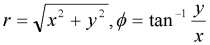Thus, our 3-step strategy for plotting any polar equation shall be as follows:

 1. Get the value of r corresponding to the various ø values (remember that ø values in polar functions are always in radians not degress). 2. Convert the polar point (r , ø) into a quadratic point (x , y). This is done by the C functions P2Q_x() and P2Q_y() in the code shown below. 3. Use the functions defined previously to plot the equation.

We shall now apply the above strategy to plot the function

r = sin(2ø) + 2 cos(3ø);

Here's the C program to do it.

Here's the result of this program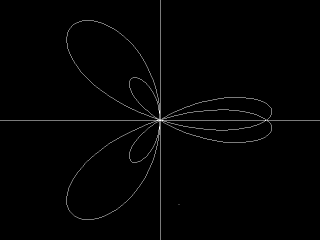If you wish to download a compiled version of this program that can run under DOS/Windows environments, click here (50 KB). You will also need this file (5.5 KB) in the same folder as the executable.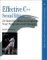Effective C++: 50 Specific Ways to Improve Your Programs and Design by Andrei Alexandrescu (Paperback)       More C/C++ books          In association with Amazon.com